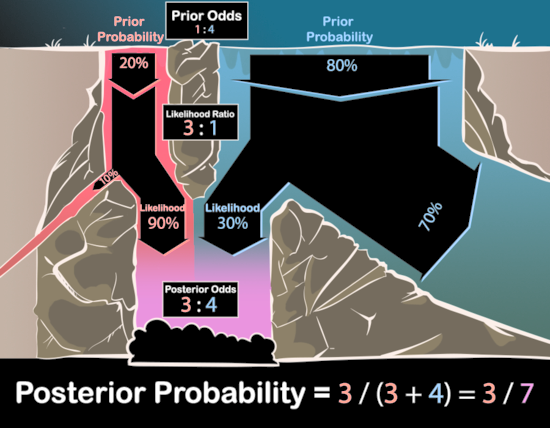# Waterfall diagram

Waterfall diagrams, like frequency diagrams, provide a way of visualizing Bayes’ Rule. Recall that Bayes’ rule (in the odds form) says that the posterior odds between any two hypotheses is equal to the prior odds times the relative likelihoods.

For example, in the Diseasitis problem, a patient is 20% likely to be sick (and 80% likely to be healthy) a priori, and they take a test that is 3x more likely to come back positive if they are sick. The odds of a patient being sick given a positive test are thus $$(1 : 4) \times (3 : 1) = (3 : 4).$$

Using waterfall diagrams, we can visualize the prior odds as two separate streams of water at the top of a waterfall, and the relative likelihoods as the proportion of water from each stream that makes it to the shared pool at the bottom. The posterior odds can then be visualized as the proportion of water in the shared pool that came from each different prior stream.See Waterfall diagrams and relative odds for a walkthrough of the diagram.

Waterfall diagrams make it clear that, when calculating the posterior odds, what matters is the relative proportion between how much each gallon of red water vs each gallon of blue water makes it into the shared pool. If 45% of red water and 15% of blue water made it to the bottom, that would give the same relative proportion of red and blue water in the shared pool at the bottom as 90% and 30%.Thus, it is only the relative likelihoods (and not the absolute likelihoods) that matter when calculating posterior odds.

Similarly, changing the water flows at the top of the waterfall from (20 gallons/​sec red water : 80 gallons/​sec blue water) to (40 gallons/​sec red water : 160 gallons/​sec blue water) would double the total water at bottom, but not change the relative proportions of blue and red water. So only the relative prior odds matter to the relative posterior odds.

Children:

Parents:

• Bayes' rule

Bayes’ rule is the core theorem of probability theory saying how to revise our beliefs when we make a new observation.

A panel within the first diagram reads:

 Posterior Odds
3:4


Impossible to see where this comes from. Revise to read:

 Posterior Odds
20% x 90% :  80% x 30%
=     0.18 : 0.24
=        3:4

• The banner reading “Your proposal has been submitted” lingers into subsequent editing processes, causing confusion.